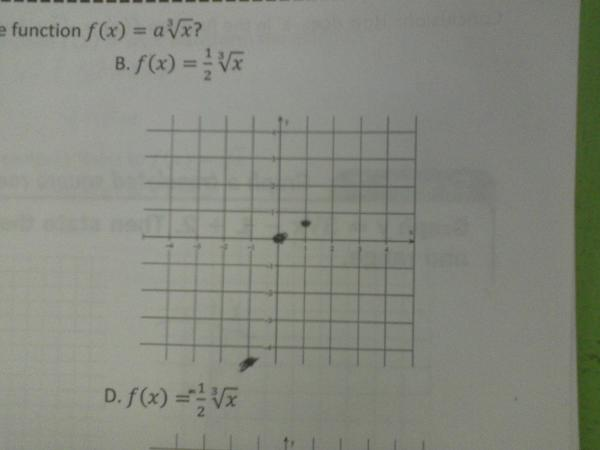Categories

# A Contest!Marshall Thompson is offering a substantial bounty for the correct explanation for this mistake. (He knows the truth from talking to the student.)

Who’s got it?

## 4 replies on “A Contest!”

Jamessays:

I think he distributed the -1 to the exponent three, giving him (in his mind), -3. He then calculated -3-2, somehow thinking he was supposed to subtract the bottom from the top, to get -5. He then multiplied that by the square root of -1 which he THOUGHT was 1, giving him -5. 🙂

Whatever number the student got was smaller than -4.5 so they just marked it on -4.5? Or they thought it was imaginary and just put it at the bottom because it has to be somewhere? Or they ended up getting -3 for the cube root of -1 and that led to 1/2 (-3) so they added 1/2 of -3 to -3 and got -4.5? I’m grasping at straws here.

For the record, I want no part of the bounty.

So if the graph is of (-1, -4.5), I do notice that -1/2 * (3^2) = -4.5. If there was a calculator involved, I could imagine a student typing 3sqrt(-1) instead of cube root, and then getting a calculator error. Maybe after 3sqrt(-1) didn’t work they thought that “negative exponents flip the fraction, and square root = 1/2 for the exponent” and turned 3sqrt(-1) into 3^2? But wouldn’t 3*(1^2) = 3 been more likely? Or if they weren’t using a calculator anymore since they got the error, why not 1^3?

Did the student use a calculator? Was this mistake consistent with how they graphed (0,0) and (1,1/2)? What happened on the following question? Was it consistent?

Max is the closest so far. This was a calculator error. For the record, the student was trying to plot the point (-1,-5).